# Maharashtra Board 12th Commerce Maths Solutions Chapter 7 Assignment Problem and Sequencing Miscellaneous Exercise 7

Balbharati Maharashtra State Board 12th Commerce Maths Solution Book Pdf Chapter 7 Assignment Problem and Sequencing Miscellaneous Exercise 7 Questions and Answers.

## Maharashtra State Board 12th Commerce Maths Solutions Chapter 7 Assignment Problem and Sequencing Miscellaneous Exercise 7

(I) Choose the correct alternative.

Question 1.
In sequencing, an optimal path is one that minimizes ___________
(a) Elapsed time
(b) Idle time
(c) Both (a) and (b)
(c) Both (a) and (b)

Question 2.
If job A to D have processing times as 5, 6, 8, 4 on first machine and 4, 7, 9, 10 on second machine then the optimal sequence is:
(a) CDAB
(b) DBCA
(c) BCDA
(d) ABCD
(b) DBCAQuestion 3.
The objective of sequence problem is
(a) to find the order in which jobs are to be made
(b) to find the time required for the completing all the job on hand
(c) to find the sequence in which jobs on hand are to be processed to minimize the total time required for processing the jobs
(d) to maximize the cost
(c) to find the sequence in which jobs on hand are to be processed to minimize the total time required for processing the jobs

Question 4.
If there are n jobs and m machines, then there will be ___________ sequences of doing the jobs.
(a) mn
(b) m(n!)
(c) nm
(d) (n!)m
(d) (n!)m

Question 5.
The Assignment Problem is solved by
(a) Simple method
(b) Hungarian method
(c) Vector method
(d) Graphical method
(b) Hungarian method

Question 6.
In solving 2 machine and n jobs sequencing problem, the following assumption is wrong
(a) No passing is allowed
(b) Processing times are known
(c) Handling times is negligible
(d) The time of passing depends on the order of machining
(d) The time of passing depends on the order of machiningQuestion 7.
To use the Hungarian method, a profit maximization assignments problem requires
(a) Converting all profit to opportunity losses
(b) A dummy person or job
(c) Matrix expansion
(d) Finding the maximum number of lines to cover all the zeros in the reduced matrix
(a) Converting all profits to opportunity losses

Question 8.
Using the Hungarian method the optimal assignment obtained for the following assignment problem to minimize the total cost is: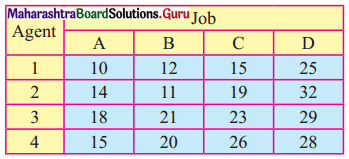(a) 1 – C, 2 – B, 3 – D, 4 – A
(b) 1 – B, 2 – C, 3 – A, 4 – D
(c) 1 – A, 2 – B, 3 – C, 4 – D
(d) 1 – D, 2 – A, 3 – B, 4 – C
(a) 1 – C, 2 – B, 3 – D, 4 – A

Question 9.
The assignment problem is said to be unbalanced if
(a) Number of rows is greater than the number of columns
(b) Number of rows is lesser than number of columns
(c) Number of rows is equal to the number of columns
(d) Both (a) and (b)
(d) Both (a) and (b)

Question 10.
The assignment problem is said to be balanced if
(a) Number of rows is greater than the number of columns
(b) Number of rows is lesser than number of columns
(c) Number of rows is equal to the number of columns
(d) If the entry of rows is zero
(c) Number of rows is equal to number of columns

Question 11.
The assignment problem is said to be balanced if it is a
(a) Square matrix
(b) Rectangular matrix
(c) Unit matrix
(d) Triangular matrix
(a) Square matrix

Question 12.
In an assignment problem if the number of rows is greater than the number of columns then
(c) Row with cost 1 is added
(d) Column with cost 1 is added

Question 13.
In a 3 machine and 5 jobs problem, the least of processing times on machines A, B, and C are 5, 1 and 3 hours and the highest processing times are 9, 5 and 7 respectively, then it can be converted to a 2 machine problem if the order of the machines is:
(a) B – A – C
(b) A – B – C
(c) C – B – A
(d) Any order
(b) A – B – CQuestion 14.
The objective of an assignment problem is to assign
(a) Number of jobs to equal number of persons at maximum cost
(b) Number of jobs to equal number of persons at minimum cost
(c) Only the maximize cost
(d) Only to minimize cost
(b) Number of jobs to equal number of persons at minimum cost

(II) Fill in the blanks.

Question 1.
An assignment problem is said to be unbalanced when ___________
the number of rows is not equal to the number of columns

Question 2.
When the number of rows is equal to the Number of columns then the problem is said to be ___________ assignment problem.
balanced

Question 3.
For solving assignment problem the matrix should be a ___________
square matrix

Question 4.
If the given matrix is not a ___________ matrix, the assignment problem is called an unbalanced problem.
square

Question 5.
A dummy row(s) or column(s) with the cost elements as ___________ the matrix of an unbalanced assignment problem as a square matrix.
zero

Question 6.
The time interval between starting the first job and completing the last, job including the idle time (if any) in a particular order by the given set of machines is called ___________
Total elapsed timeQuestion 7.
The time for which a machine j does not have a job to process to the start of job i is called ___________
Idle time

Question 8.
The maximization assignment problem is transformed to minimization problem by subtracting each entry in the table from the ___________ value in the table.
maximum

Question 9.
When the assignment problem has more than one solution, then it is ___________ optimal solution.
multiple

Question 10.
The time required for printing four books A, B, C, and D is 5, 8, 10, and 7 hours. While its data entry requires 7, 4, 3, and 6 hrs respectively. The sequence that minimizes total elapsed time is ___________
A – D – B – C

(III) State whether each of the following is True or False.

Question 1.
One machine – one job is not an assumption in solving sequencing problems.
False

Question 2.
If there are two least processing times for machine A and machine B, priority is given for the processing time which has the lowest time of the adjacent machine.
True

Question 3.
To convert the assignment problem into a maximization problem, the smallest element in the matrix is deducted from all other elements.
False

Question 4.
The Hungarian method operates on the principle of matrix reduction, whereby the cost table is reduced to a set of opportunity costs.
TrueQuestion 5.
In a sequencing problem, the processing times are dependent on the order of processing the jobs on machines.
False

Question 6.
The optimal assignment is made in the Hungarian method to cells in the reduced matrix that contain a Zero.
True

Question 7.
Using the Hungarian method, the optimal solution to an assignment problem is fund when the minimum number of lines required to cover the zero cells in the reduced matrix equals the number of people.
True

Question 8.
In an assignment problem, if a number of columns are greater than the number of rows, then a dummy column is added.
False

Question 9.
The purpose of a dummy row or column in an assignment problem is to obtain a balance between a total number of activities and a total number of resources.
True

Question 10.
One of the assumptions made while sequencing n jobs on 2 machines is: two jobs must be loaded at a time on any machine.
False

(IV) Solve the following problems.

Part – I

Question 1.
A plant manager has four subordinates, and four tasks to be performed. The subordinates differ in efficiency and the tasks differ in their intrinsic difficulty. This estimate of the times each man would take to perform each task is given in the effectiveness matrix below.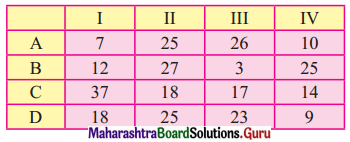How should the tasks be allocated, one to a man, as to minimize the total man-hours?
Solution:
The hr matrix is given by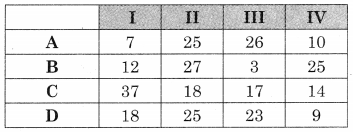Subtracting row minimum from all values in that row we get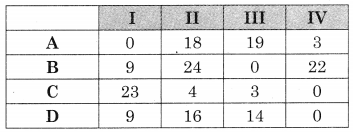Subtracting column minimum from all values in that column we get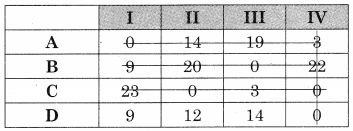The minimum no. of lines covering ail the zeros (4) is equal to the order of the matrix (4)
∴ The assignment is possible.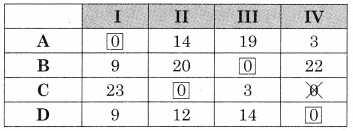The assignment is
A → I, B → III, C → II, D → IV
For the minimum hrs. take the corresponding value from the hr matrix.
Minimum hrs = 7 + 3 + 18 + 9 = 37 hrsQuestion 2.
A dairy plant has five milk tankers, I, II, III, IV & V. These milk tankers are to be used on five delivery routes A, B, C, D & E. The distances (in kms) between the dairy plant and the delivery routes are given in the following distance matrix.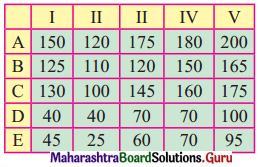How should the milk tankers be assigned to the chilling centre so as to minimize the distance travelled?
Solution:
The distance matrix is given by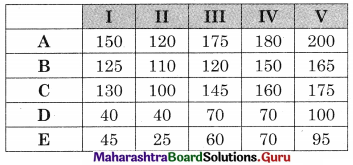Subtracting row minimum from all values in that row we get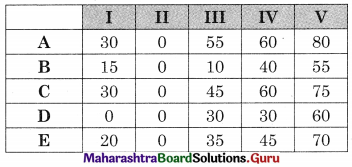Subtracting column minimum from each value in that column we get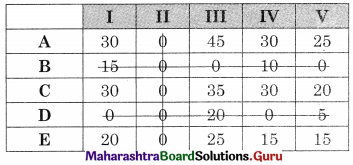The number of lines covering all the zeros (3) is less than the order of the matrix (5) so the assignment is not possible. The modification is required.
The minimum uncovered value (15) is subtracted from uncovered values and added to the values at the intersection. The numbers on the lines remain the same. We get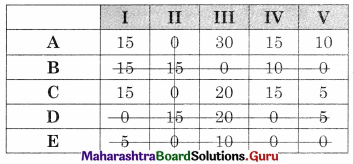The minimum lines covering all the zeros (4) are less than the order of the matrix (5) so the assignment is not possible. The modification is required the minimum uncovered value (5) is subtracted from uncovered values and added to the values at the intersection. The numbers on the lines remain the same we get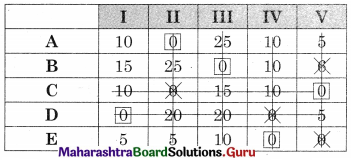The minimum number of lines covering all the zeros (5) is equal to the order of the matrix (5) So assignment is possible.
The assignment is
A → II, B → III, C → V, D → I, E → IV
Total minimum distance is = 120 + 120 + 175 + 40 + 70 = 525 kms.

Question 3.
Solve the following assignment problem to maximize sales: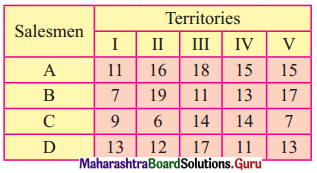Solution:
As it is a maximization problem so we need to convert it into a minimization problem.
Subtracting all the values from the maximum value (19) we get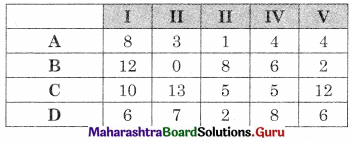Also, it is an unbalanced problem so we need to add a dummy row (E) with all values zero, we get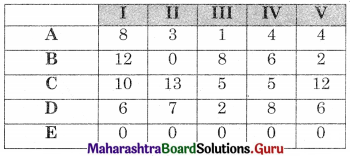Subtracting row minimum from all values in that row we get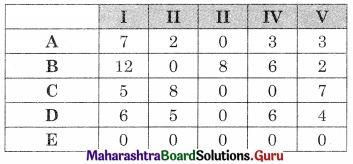Subtracting column minimum from all values in that column we get the same matrix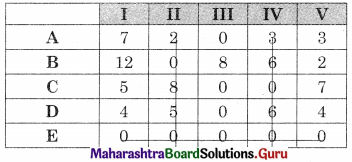The minimum number of lines covering all the zero (4) is less than the order of the matrix (5) So assignment is not possible. The modification is required. The minimum uncovered value (2) is subtracted from the uncovered values and added to the values at the intersection. The values on the lines remain the same. We get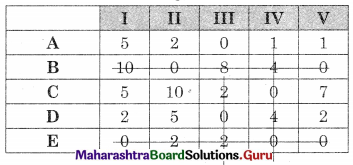The minimum number of lines covering all the zeros (4) is less than the order of the matrix (5) so the assignment is not possible. The modification is required. The minimum uncovered value (1) is subtracted from the uncovered value and added to the values at the intersection. The values on the lines remain the same. We get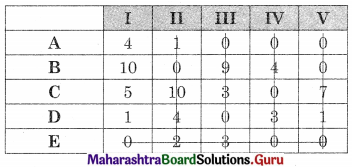The minimum number of lines covering all the zeros (5) is equal to the order of the matrix (5) so the assignment is possible.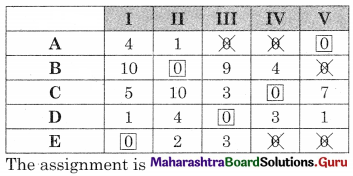The assignment is
A → V, B → II, C → IV, D → III, E → I
No salesman goes to I as E is a dummy row.
For the maximum value take the corresponding values from the original matrix.
We get Maximum value = 15 + 19 + 14 + 17 + 0 = 65 unitsQuestion 4.
The estimated sales (tons) per month in four different cities by five different managers are given below: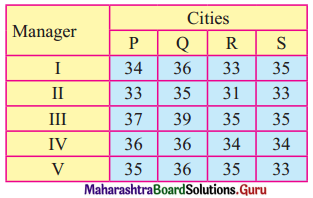Find out the assignment of managers to cities in order to maximize sales.
Solution:
This is a maximizing problem. To convert it into minimizing problem subtract all the values of the matrix from the maximum (largest) value (39) we get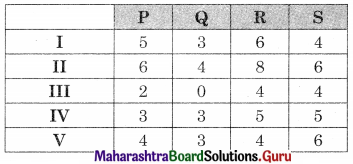Also as it is an unbalanced problem so we have to add a dummy column (T) with all the values as zero. We get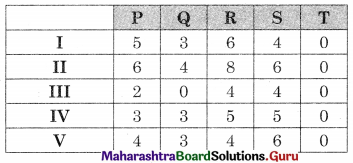Subtracting row minimum from all values in that row we get the same matrix
Subtracting column minimum from all values in that column we get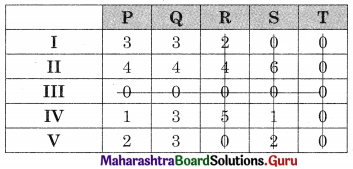The minimum number of lines covering all the zeros (4) is less than the order of the matrix (5) so assignments are not possible. The modification is required. The minimum uncovered value (1) is subtracted from the uncovered values and added to the values at the intersection. The values on the lines remain the same. We get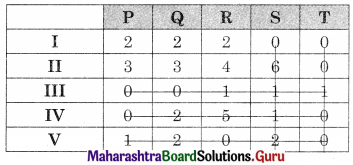The minimum number of lines covering all the zeros (5) is equal to the order of the matrix (5) so the assignment is possible.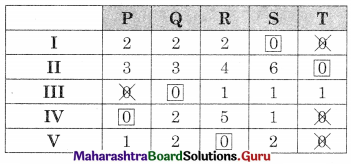So I → S, II → T, III → Q, IV → P, V → R.
As T is dummy manager II is not given any city.
To find the maximum sales we take the corresponding value from the original matrix
Total maximum sales = 35 + 39 + 36 + 35 = 145 tons

Question 5.
Consider the problem of assigning five operators to five machines. The assignment costs are given in the following table.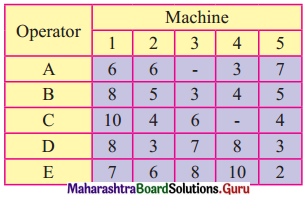Operator A cannot be assigned to machine 3 and operator C cannot be assigned to machine 4. Find the optimal assignment schedule.
Solution:
This is a restricted assignment problem, so we assign a very high cost (oo) to the prohibited cells we get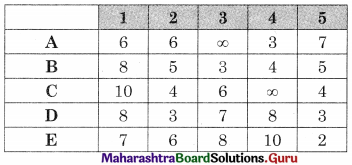Subtracting row minimum from all values in that row we get.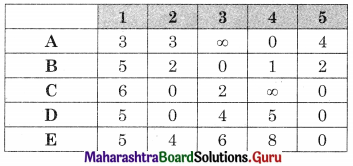Subtracting column minimum from all values in that column we get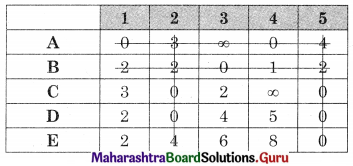As the minimum number of lines covering all the zeros (4) is equal to the order of the matrix (5) so the assignment is not possible. The modification is required. The minimum uncovered value (2) is subtracted from all the uncovered values and added to the values at the intersection. The values on the lines remain the same. We get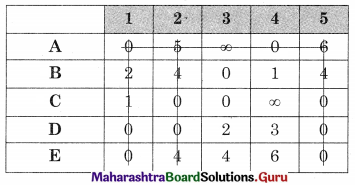As the minimum number of lines covering all the zeros (5) is equal to the order of the matrix, assignment is the possible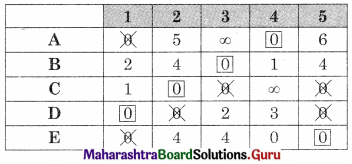So A → 4, B → 3, C → 2, D → 1, E → 5
For the minimum cost take the corresponding values from the cost matrix we get
Total minimum cost = 3 + 3 + 4 + 3 + 7 = 20 unitsQuestion 6.
A chartered accountant’s firm has accepted five new cases. The estimated number of days required by each of their five employees for each case are given below, where-means that the particular employee can not be assigned the particular case. Determine the optimal assignment of cases of the employees so that the total number of days required to complete these five cases will be minimum. Also, find the minimum number of days.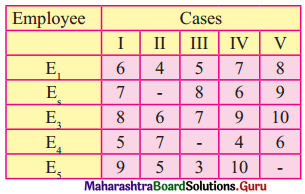Solution:
This is a restricted assignment problem so we assign a very high cost (∞) to all the prohibited cells. The day matrix becomes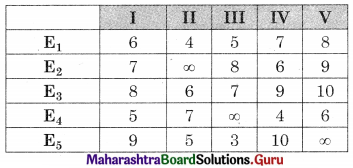Subtracting row minimum from all values in that row we get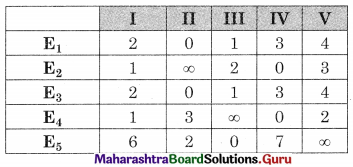Subtracting column minimum from all values in that column we get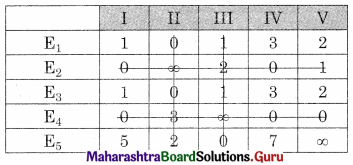The minimum number of lines covering all the zeros (4) is less than the order of the matrix (5) so the assignment is not possible, The modification is required. The minimum uncovered value (1) is subtracted from all the uncovered values and added to the values at the intersection. The values on the lines remain the same, we get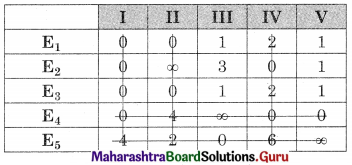The minimum number of lines covering all the zeros (5) is equal to the order of the matrix (5) so the assignment is possible.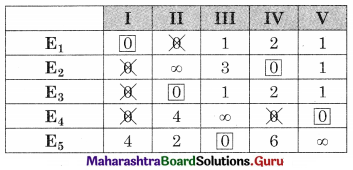So E1 → I, E2 → IV, E3 → II, E4 → V, E5 → III
To find the minimum number of days we take the corresponding values from the day matrix.
Total minimum number of days = 6 + 6 + 6 + 6 + 3 = 27 days

Part – II

Question 1.
A readymade garments manufacture has to process 7 items through two stages of production, namely cutting and sewing. The time taken in hours for each of these items in different stages are given below: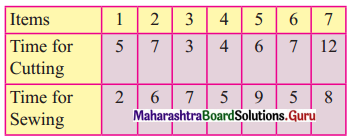Find the sequence in which these items are to be processed through these stages so as to minimize the total processing time. Also, find the idle time of each machine.
Solution:
Let A = cutting and B = sewing. So we have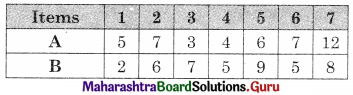Observe min {A, B} = 2 for item 1 for B.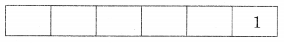The problem reduces to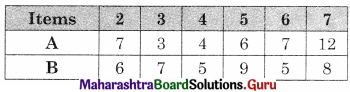Now min {A, B} = 3 for item 3 for A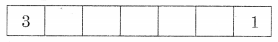The problem reduces to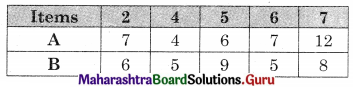New min {A , B} = 4 for item 4 for A.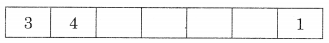The problem reduces to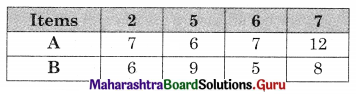Now min(A, B} = 5 for item 6 for B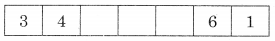The problem reduces to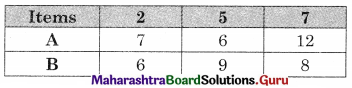Now min {A, B} = 6 for item 5 for A and item 2 for B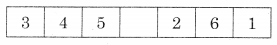Now only 7 is left
∴ The optimal sequence is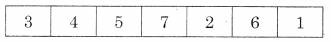Worktable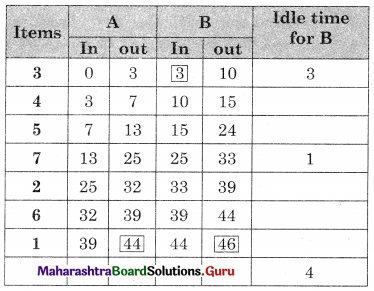Total elapsed time = 46 hrs
Idle time for A (cutting) = 46 – 44 = 2 hrs
Idle time for B (Sewing) = 4 hrsQuestion 2.
Five jobs must pass through a lathe and a surface grinder, in that order. The processing times in hours are shown below. Determine the optimal sequence of the jobs. Also, find the idle time of each machine.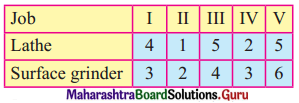Solution:
Let A = lathe and B = surface grinder. We have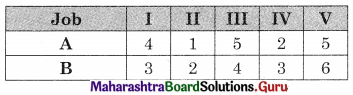Observe min {A, B} = 1 for job II for A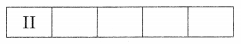The problem reduces to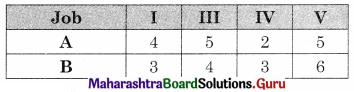Now min {A, B} = 2 for job IV for A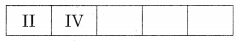The problem reduces to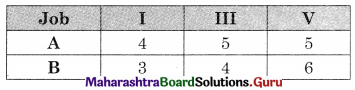Now min {A, B} = 3 for job I for B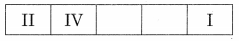The problem reduces to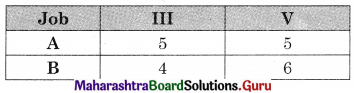Now min {A, B} = 5 for jobs III and V for A
∴ We have two options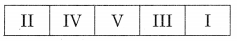or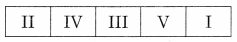We take the first one.
Worktable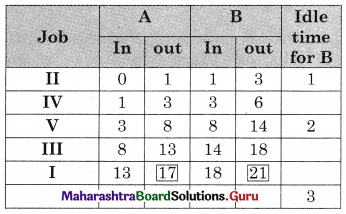Total elapsed time = 21 hrs
Idle time for A (lathe) = 21 – 17 = 4 hrs
Idle time for B (surface grinder) = 3 hrs

Question 3.
Find the sequence that minimizes the total elapsed time to complete the following jobs. Each job is processed in order AB.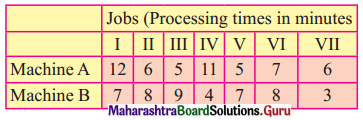Determine the sequence for the jobs so as to minimize the processing time. Find the total elapsed time and the idle time for both machines.
Solution:
Observe min {A, B} = 3 for job VII on B.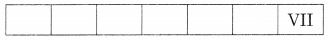The problem reduces to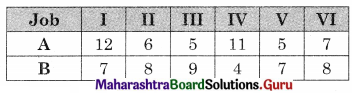Now min {A, B} = 4 for job IV on B.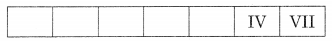The problem reduces to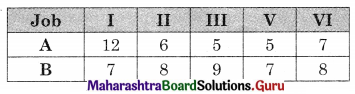Now min {A, B} = 5 for job III & V on A. we have two options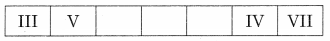or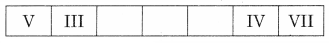We take the first one
The problem reduces to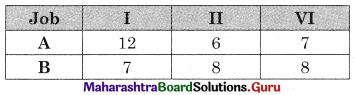Now min {A, B} = 5 for job II on A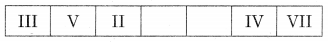The problem reduces to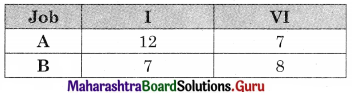Now min {A, B} = 7 for a job I on B and for job VI on A
∴ The optional sequence is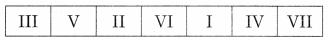Worktable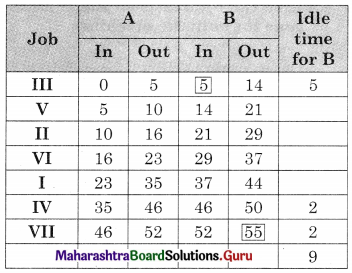Total elapsed time = 55 units
Idle time for A = 55 – 52 = 3 units
Idle time for B = 9 units.Question 4.
A toy manufacturing company has five types of toys. Each toy has to go through three machines A, B, C in the order ABC. The time required in hours for each process is given in the following table.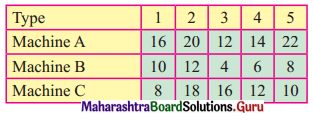Solve the problem for minimizing the total elapsed time.
Solution:
Min A = 12, Max B = 12
As min A ≥ max B.
The problem can be converted into two machine problems.
Let G and H be two fictitious machines such that G = A + B and H = B + C, We get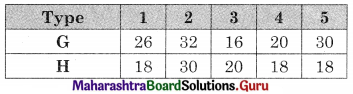Now min {G, H} = 16 for type 3 on G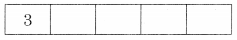The problem reduces to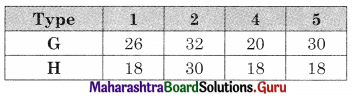Min (G, H} = 18 for type 1, 4 & 5 on H
We have more than one option, we take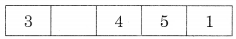Now only type 2 is left.
∴ The optional sequence is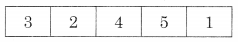Worktable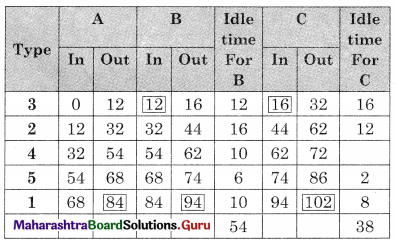Total elapsed time = 102 hours
Idle time for A = 102 – 84 = 18 hours
Idle time for B = 54 + (102 – 94) = 62 hours
Idle time for C = 38 hours

Question 5.
A foreman wants to process 4 different jobs on three machines: a shaping machine, a drilling machine, and a tapping, the sequence of operations being shaping-drilling-tapping. Decide the optimal sequence for the four jobs to minimize the total elapsed time. Also, find the total elapsed time and the idle time for every machine.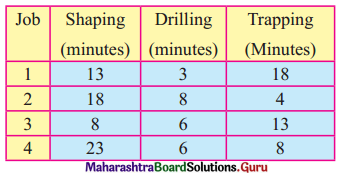Solution:
The time matrix is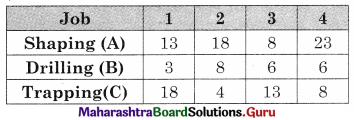Min A = 8, Max B = 8, as min A ≥ max B.
The problem can be converted into a two-machine problem.
Let G and H be two fictitious machines such that
G = A + B and H = B + C we get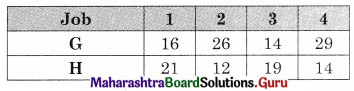Observe min (G, H} = 12 for job 2 on H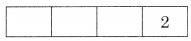The problem reduces to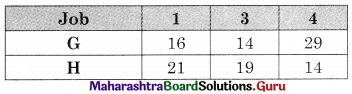Now min {G, H} = 14 for job 3 on G and job 4 on H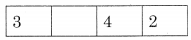Now only job 1 is left.
∴ The optimal sequence is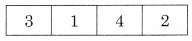Worktable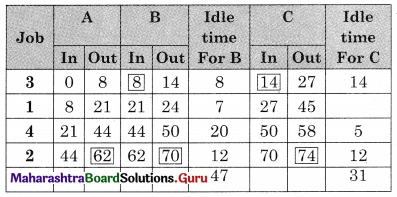Total elapsed time = 74 min
Idle time for A (shapping) = 74 – 62 = 12 min
Idle time for B (Drilling) = 47 + (74 – 70) = 51 min
Idle time for C (trapping) = 31 min